# Triangle Problems

#### Number of problems found: 1272

• Isosceles trapezoidFind the height in an isosceles trapezoid if the area is 520 cm2 and the base a = 25 cm and c = 14 cm. Calculate the interior angles of the trapezoid.
• Length of the chord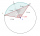Calculate the length of the chord in a circle with a radius of 25 cm with a central angle of 26°.
• A rectangle 5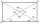A rectangle has sides of 10 cm and 14 cm. Calculate the angle between a diagonal and a long side.
• JoanneJoanne and Roger are planting a rectangular garden. The garden is 8 1/2 ft by 13 ft. They want to use half of the garden for cucumbers and half of the garden for tomatoes. They decide to separate the garden into two right triangles. What is the area of th
• School modelThe beech school model of a regular quadrilateral pyramid has a base 20 cm long and 24 cm high. Calculate a) the surface of the pyramid in square decimeters, b) the mass of the pyramid in kilograms if the density of the beech is ρ = 0,8 g/cm ^ 3
• Parallelogram ABCDWe have the parallelogram ABCD, where AB is 6.2 cm BC is 5.4 cm AC is 4.8 cm calculate the height on the AB side and the angle DAB
• The rectangularThe rectangular trapezoid has bases 15 dm and 8 dm long and the length of the inclined arm is 12 dm. How long is the other arm of the trapezoid?
• The triangleThe triangle has sides 5 cm long, 5 cm, 8 cm long. What is the area of the triangle?
• Right-angled triangle baseFind the volume and surface area of a triangular prism with a right-angled triangle base if the length of the prism base legs are 7.2 cm and 4.7 cm and the height of a prism is 24 cm.
• Goat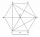The fenced flower bed has the shape of a regular hexagon. The tops are formed by fence posts. The fence around the flowerbed measures 60 m. A goat is tied to one of the pillars from the outside and grazes on the surrounding meadow (the goat should not ent
• How toHow to find a total surface of a rectangular pyramid if each face is to be 8 dm high and the base is 10 dm by 6 dm.
• Calculate triangleIn the triangle ABC, calculate the sizes of all heights, angles, perimeters and its area, if given a-40cm, b-57cm, c-59cm
• Frustrum - volume, areaCalculate the surface and volume of the truncated cone, the radius of the smaller figure is 4 cm, the height of the cone is 4 cm and the side of the truncated cone is 5 cm.
• Main/central vertex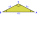ABC is an isosceles triangle with base BC and main vertex A. The angle at vertex A is 18°. What will be the size of the angle at vertex B?
• Sin cos tan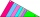In triangle ABC, right-angled at B. Sides/AB/=7cm, /BC/=5cm, /AC/=8.6cm. Find to two decimal places. A. Sine C B. Cosine C C. Tangent C.
• CalculateCalculate the height to the base of the isosceles triangle ABC if the length of the base is c = 24cm and the arms have a length b = 13cm.
• Find theFind the third interior angle of the triangle ABC where: α = 48°, γ = 65°.
• Rectangular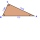Rectangular triangle KLM with right angle at vertex L, angle beta at vertex K and angle alpha at vertex M. Angle at vertex M = 65°, side l = 17.5 cm. Use Pythagorean theorems and trigonometric functions to calculate the lengths of all sides and the angle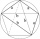The quadrilateral ABCD is composed of two right triangles ABD and BCD. For side lengths: |AD| = 3cm, | BC | = 12cm, | BD | = 5cm. How many square centimeters (area) does the quadrilateral ABCD have? The angles DAB and DBC are right.
• Divide an isosceles triangleHow to divide an isosceles triangle into two parts with equal contents perpendicular to the axis of symmetry (into a trapezoid and a triangle)?

Do you have an exciting math question or word problem that you can't solve? Ask a question or post a math problem, and we can try to solve it.

We will send a solution to your e-mail address. Solved examples are also published here. Please enter the e-mail correctly and check whether you don't have a full mailbox.

Please do not submit problems from current active competitions such as Mathematical Olympiad, correspondence seminars etc...